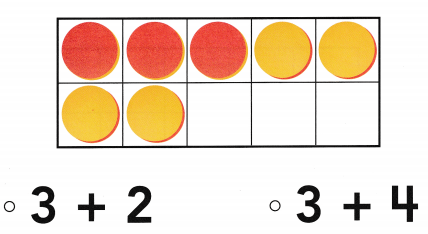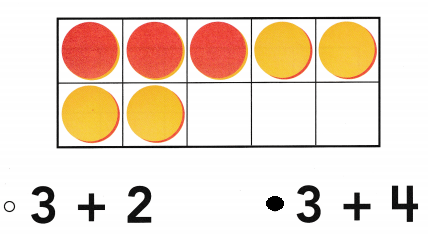# Texas Go Math Kindergarten Lesson 10.1 Answer Key Compose 6 and 7

Refer to our Texas Go Math Kindergarten Answer Key Pdf to score good marks in the exams. Test yourself by practicing the problems from Texas Go Math Kindergarten Lesson 10.1 Answer Key Compose 6 and 7.

## Texas Go Math Kindergarten Lesson 10.1 Answer Key Compose 6 and 7

Essential Question
How do you put together numbers to make 6 and 7?

Explore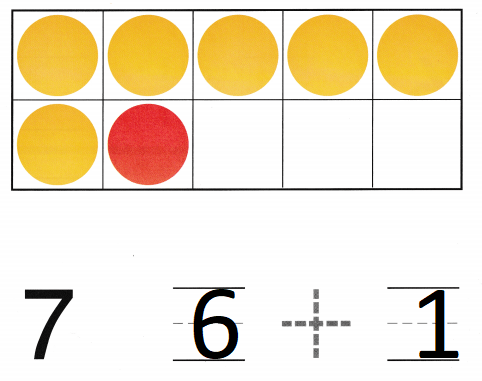Explanation:
There are 6 yellow dots and 1 red dot
6 + 1 = 7

Directions
Place yellow and red counters in the ten frame as shown. Write the numbers and te the symbol to show the sets that are put together.

Share and Show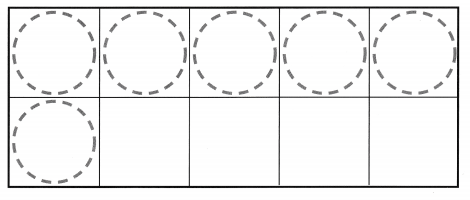Question 1.Explanation:
The number of red counters are 3
the number of yellow counters are 3
the total number of counters are 6
3 + 3 = 6

Question 2.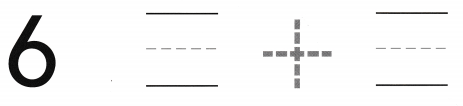Explanation:
The number of red counters are 2
the number of yellow counters are 4
the total number of counters are 6
4 + 2 = 6

Question 3.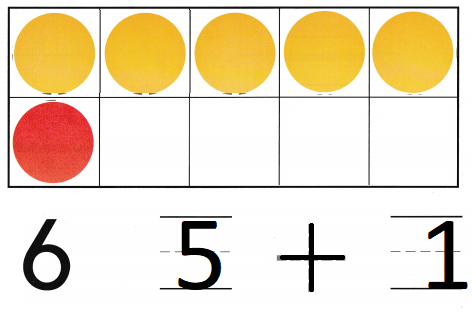Explanation:
The number of red counters are 1
the number of yellow counters are 5
the total number of counters are 6
5 + 1 = 6

Directions
1-2. Place counters in the ten frame to model numbers put together to make 6. How many are there of each color counter? Write the numbers and trace the symbol. 3. Place counters in the ten frame to model numbers put together to make 6. Write the numbers for each color and trace the symbol. Draw and color the counters to match the numbers.Question 4.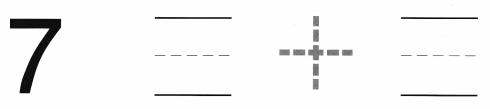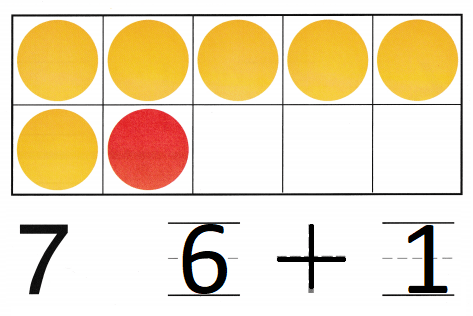Explanation:
The number of red counters are 1
the number of yellow counters are 6
the total number of counters are 7
6 + 1 = 7

Question 5.Explanation:
The number of red counters are 2
the number of yellow counters are 5
the total number of counters are 7
5 + 2 = 7

Question 6.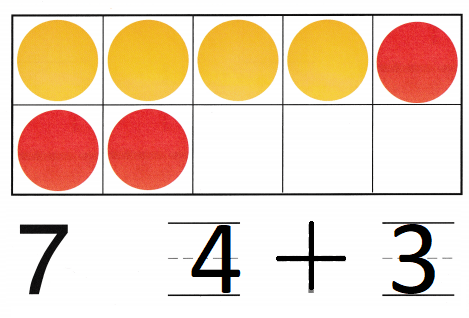Explanation:
The number of red counters are 3
the number of yellow counters are 4
the total number of counters are 7
4 + 3 = 7

Directions
1-5. Place counters in the ten frame to model numbers put together to make 7. Write the numbers and trace the symbol. 6. Draw and color counters to model numbers put together to make 7. Write the numbers and trace the symbol.

Problem Solving

Question 7.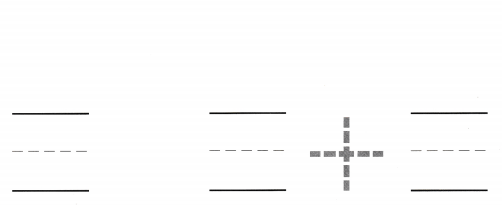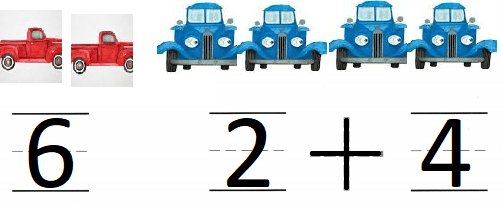Explanation:
2 red trucks and 4 blue trucks
so, in all there are 6 trucks.

Question 8.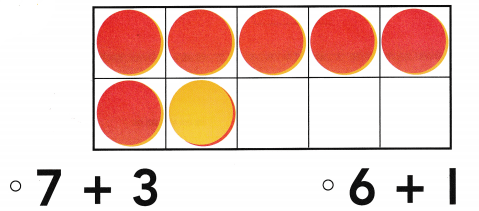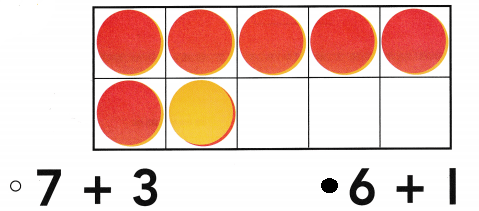Explanation:
6 red counters and 1 yellow counter
so, in all there are 7 counters
6 + 1 = 7

Directions
7. Tray has some toy trucks. Two of the trucks are red, Write the number and trace the symbol. Four of the trucks are blue. Write the number, How many trucks does he have? Draw to solve the problem. Write the number. 8. Choose the correct answer. Count the counters. Which numbers are put together?

### Texas Go Math Kindergarten Lesson 10.1 Homework and Practice

Question 1.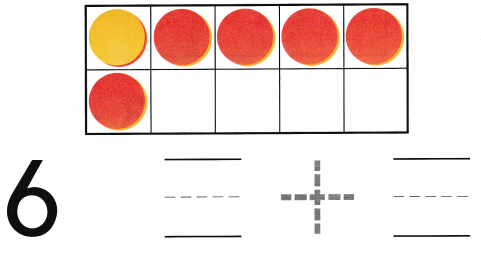Explanation:
The number of red counters are 5
the number of yellow counters are 1
the total number of counters are 6
1 + 5 = 6

Question 2.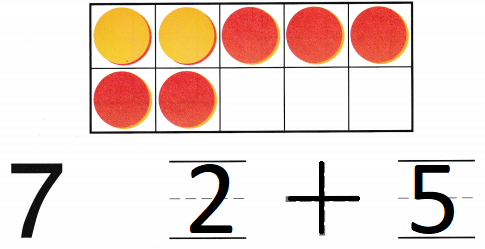Explanation:
The number of red counters are 5
the number of yellow counters are 2
the total number of counters are 7
5 + 2 = 7

Directions
1-2. Count the counters in the ten frame that model numbers put together. How many are there of each color counter? Write the numbers and trace the symbol.

Texas Test Prep

Lesson Check

Question 3.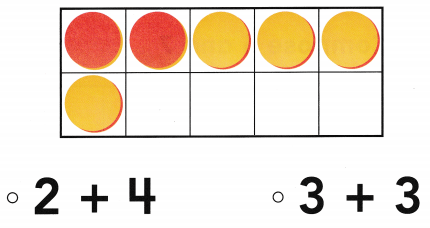Explanation:
There are 2 red counters
There are 4 yellow counters
2 + 4 = 6

Question 4.